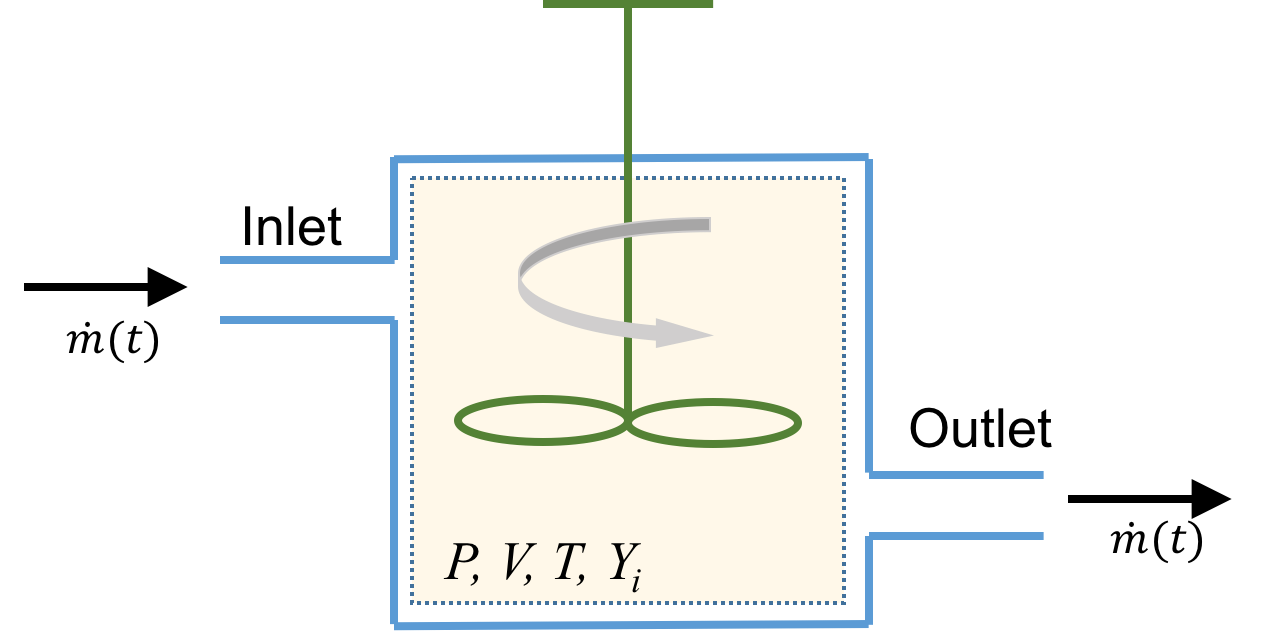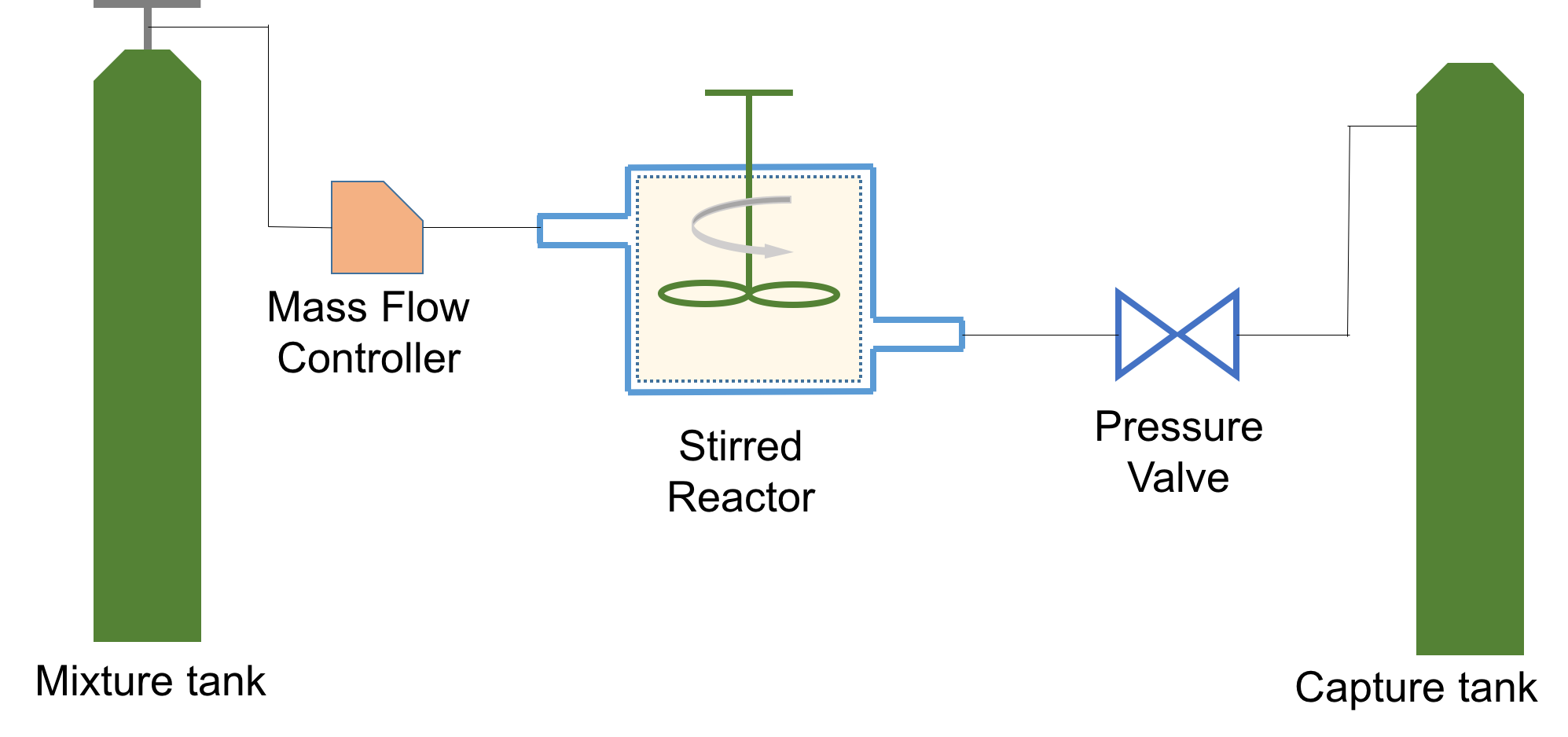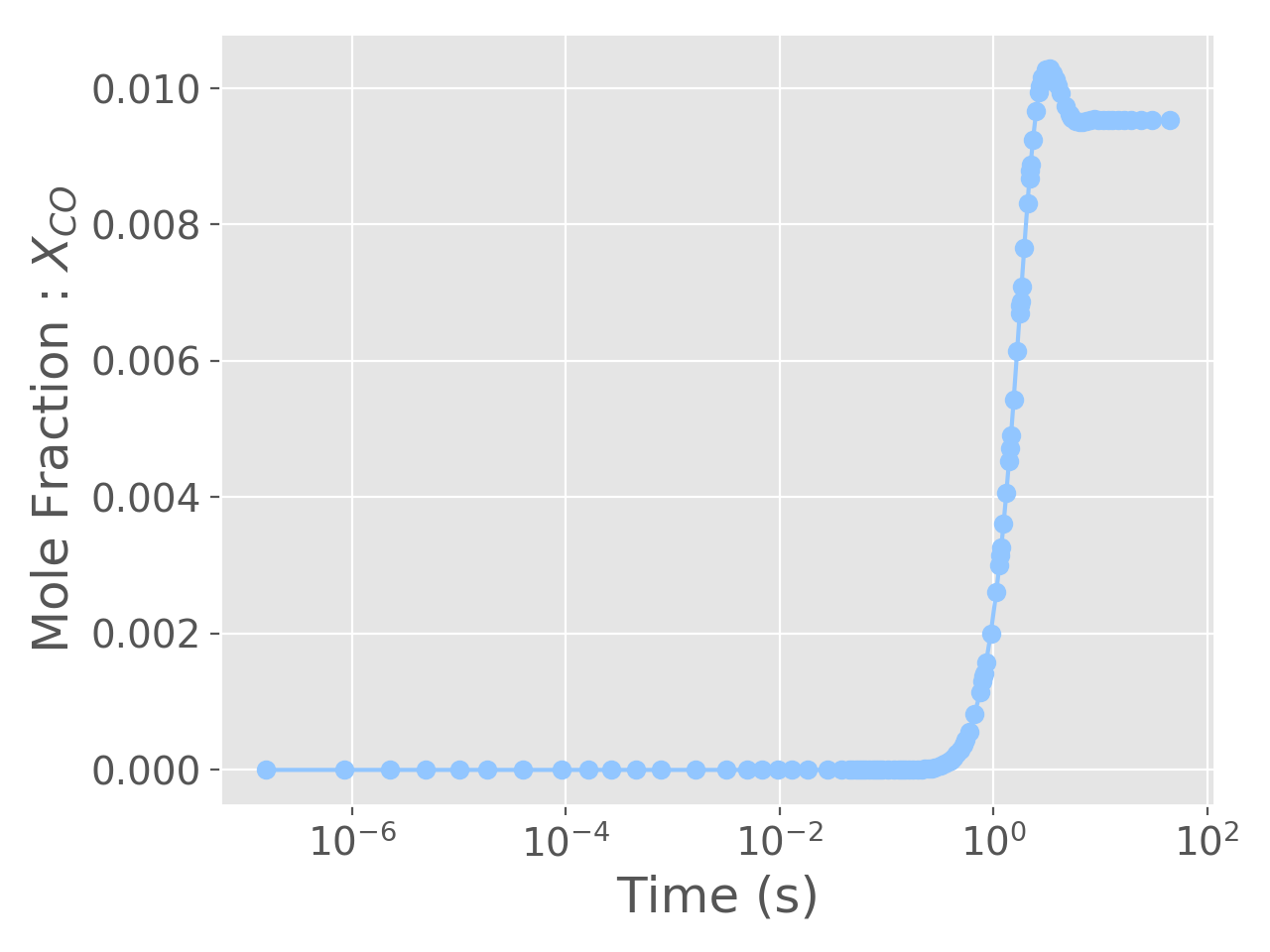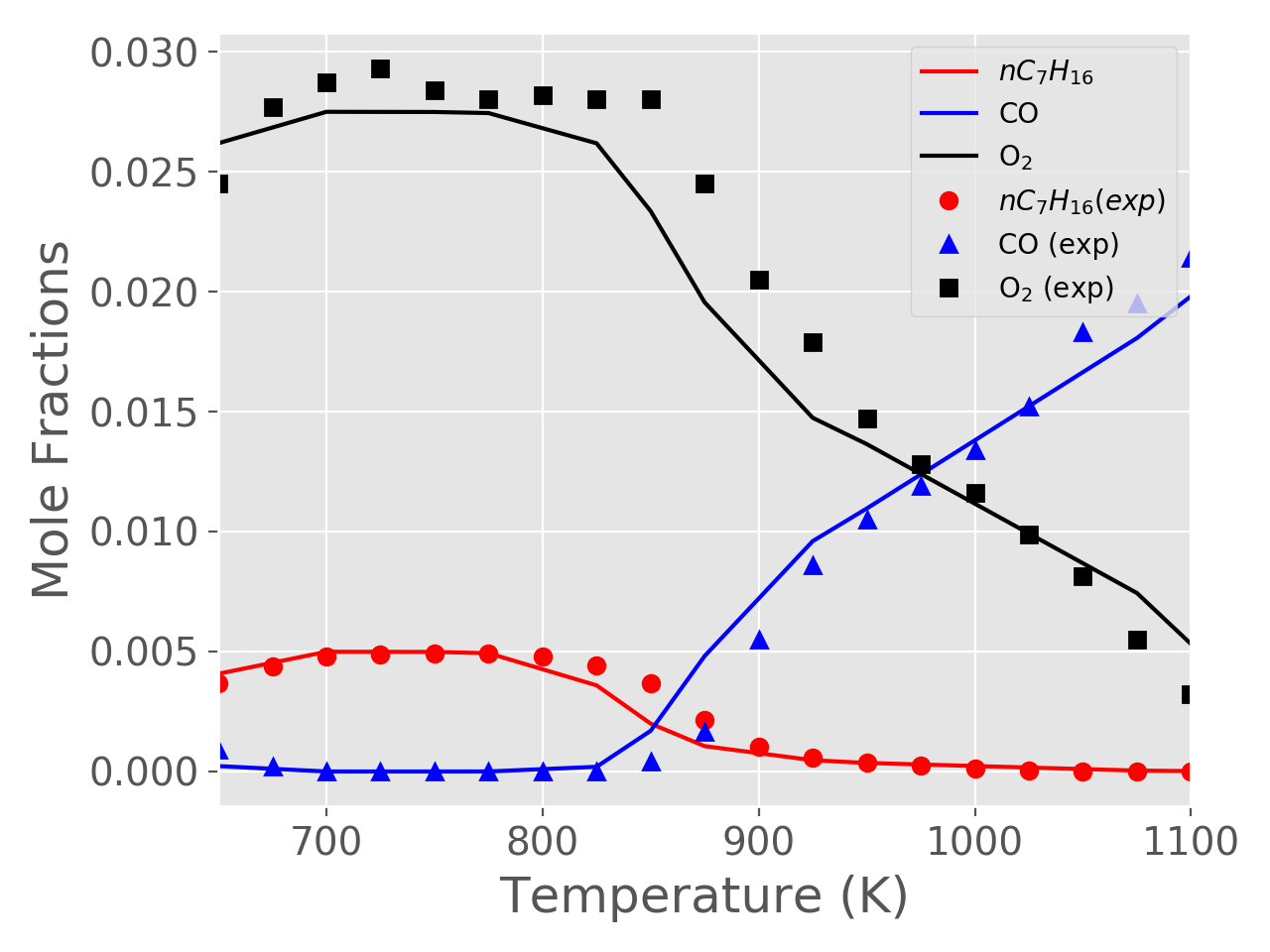# stirred_reactor.ipynb (Source)

## Continuous Reactor Example¶

### Simulation of a CSTR/PSR/WSR¶

In this example we will illustrate how Cantera can be used to simulate a Continuously Stirred Tank Reactor (CSTR), also interchangeably referred to as a Perfectly Stirred Reactor or a Well Stirred Reactor, a Jet Stirred Reactor or a Longwell Reactor (there may well be more "aliases"). A cartoon of such a reactor is shown belowAs the figure illustrates, this is an open system (unlike a Batch Reactor which is isolated). P, V and T are the reactor's pressure, volume and temperature respectively. The mass flow rate at which reactants come in is the same as that of the products which exit; and these stay in the reactor for a characteristic time $\tau$, called the residence time. This is a key quantity in sizing the reactor and is defined as follows:

\begin{equation*} \tau = \frac{m}{\dot{m}} \end{equation*}

where $m$ is the mass of the gas

In :
from __future__ import division
from __future__ import print_function

import pandas as pd
import numpy as np
import time
import cantera as ct

print("Running Cantera version: {}".format(ct.__version__))

Running Cantera version: 2.5.0a2


### Import modules and set plotting defaults¶

In :
%matplotlib notebook
import matplotlib.pyplot as plt

plt.style.use('ggplot')
plt.style.use('seaborn-pastel')

plt.rcParams['axes.labelsize'] = 18
plt.rcParams['xtick.labelsize'] = 14
plt.rcParams['ytick.labelsize'] = 14
plt.rcParams['figure.autolayout'] = True


### Define the gas¶

In this example, we will work with $nC _{7}H_{16}$/$O_{2}$/$He$ mixtures, for which experimental data can be found in the paper by Zhang et al.. We will use the same mechanism reported in the paper. It consists of 1268 species and 5336 reactions

In :
gas = ct.Solution('data/galway.cti')


**** WARNING ****
For species OHV, discontinuity in h/RT detected at Tmid = 1000
Value computed using low-temperature polynomial:  53.6206
Value computed using high-temperature polynomial: 53.5842

**** WARNING ****
For species CHV, discontinuity in h/RT detected at Tmid = 1000
Value computed using low-temperature polynomial:  107.505
Value computed using high-temperature polynomial: 107.348


### Define initial conditions¶

#### Inlet conditions for the gas and reactor parameters¶

In :
# Inlet gas conditions
reactorTemperature = 925  # Kelvin
reactorPressure = 1.046138*ct.one_atm  # in atm. This equals 1.06 bars
concentrations = {'NC7H16': 0.005, 'O2': 0.0275, 'HE': 0.9675}
gas.TPX = reactorTemperature, reactorPressure, concentrations

# Reactor parameters
residenceTime = 2  # s
reactorVolume = 30.5*(1e-2)**3  # m3

# Instrument parameters

# This is the "conductance" of the pressure valve and will determine its efficiency in
# holding the reactor pressure to the desired conditions.
pressureValveCoefficient = 0.01

# This parameter will allow you to decide if the valve's conductance is acceptable. If there
# is a pressure rise in the reactor beyond this tolerance, you will get a warning
maxPressureRiseAllowed = 0.01


#### Simulation parameters¶

In :
# Simulation termination criterion
maxSimulationTime = 50  # seconds


### Reactor arrangement¶

We showed a cartoon of the reactor in the first figure in this notebook; but to actually simulate that, we need a few peripherals. A mass-flow controller upstream of the stirred reactor will allow us to flow gases in, and in-turn, a "reservoir" which simulates a gas tank is required to supply gases to the mass flow controller. Downstream of the reactor, we install a pressure regulator which allows the reactor pressure to stay within. Downstream of the regulator we will need another reservoir which acts like a "sink" or capture tank to capture all exhaust gases (even our simulations are environmentally friendly !). This arrangment is illustrated below#### Initialize the stirred reactor and connect all peripherals¶

In :
fuelAirMixtureTank = ct.Reservoir(gas)
exhaust = ct.Reservoir(gas)

stirredReactor = ct.IdealGasReactor(gas, energy='off', volume=reactorVolume)

massFlowController = ct.MassFlowController(upstream=fuelAirMixtureTank,
downstream=stirredReactor,
mdot=stirredReactor.mass/residenceTime)

pressureRegulator = ct.Valve(upstream=stirredReactor,
downstream=exhaust,
K=pressureValveCoefficient)

reactorNetwork = ct.ReactorNet([stirredReactor])

In :
# Now compile a list of all variables for which we will store data
columnNames = [stirredReactor.component_name(item) for item in range(stirredReactor.n_vars)]
columnNames = ['pressure'] + columnNames

# Use the above list to create a DataFrame
timeHistory = pd.DataFrame(columns=columnNames)

In :
# Start the stopwatch
tic = time.time()

# Set simulation start time to zero
t = 0
counter = 1
while t < maxSimulationTime:
t = reactorNetwork.step()

# We will store only every 10th value. Remember, we have 1200+ species, so there will be
# 1200 columns for us to work with
if(counter%10 == 0):
# Extract the state of the reactor
state = np.hstack([stirredReactor.thermo.P, stirredReactor.mass,
stirredReactor.volume, stirredReactor.T, stirredReactor.thermo.X])

# Update the dataframe
timeHistory.loc[t] = state

counter += 1

# Stop the stopwatch
toc = time.time()

print('Simulation Took {:3.2f}s to compute, with {} steps'.format(toc-tic, counter))

# We now check to see if the pressure rise during the simulation, a.k.a the pressure valve
# was okay
pressureDifferential = timeHistory['pressure'].max()-timeHistory['pressure'].min()
if(abs(pressureDifferential/reactorPressure) > maxPressureRiseAllowed):
print("WARNING: Non-trivial pressure rise in the reactor. Adjust K value in valve")

Simulation Took 8.79s to compute, with 1153 steps


## Plot the results¶

As a test, we plot the mole fraction of $CO$ and see if the simulation has converged. If not, go back and adjust max. number of steps and/or simulation time

In :
plt.figure()
plt.semilogx(timeHistory.index, timeHistory['CO'],'-o')
plt.xlabel('Time (s)')
plt.ylabel(r'Mole Fraction : $X_{CO}$');## Illustration : Modeling experimental data¶

### Let us see if the reactor can reproduce actual experimental measurements¶

We first load the data. This is also supplied in the paper by Zhang et al. as an excel sheet

In :
expData = pd.read_csv('data/zhangExpData.csv')

Out:
T NC7H16 O2 CO CO2
0 500 0.00507 0.0293 0.000000 0.000000
1 525 0.00492 0.0286 0.000000 0.000000
2 550 0.00466 0.0285 0.000000 0.000000
3 575 0.00416 0.0263 0.000243 0.000101
4 600 0.00355 0.0233 0.000968 0.000251
In :
# Define all the temperatures at which we will run simulations. These should overlap
# with the values reported in the paper as much as possible
T = [650, 700, 750, 775, 825, 850, 875, 925, 950, 1075, 1100]

# Create a DataFrame to store values for the above points
tempDependence = pd.DataFrame(columns=timeHistory.columns)
tempDependence.index.name = 'Temperature'


Now we simply run the reactor code we used above for each temperature

In :
inletConcentrations = {'NC7H16': 0.005, 'O2': 0.0275, 'HE': 0.9675}
concentrations = inletConcentrations

for temperature in T:
# Re-initialize the gas
reactorTemperature = temperature  # Kelvin
reactorPressure = 1.046138*ct.one_atm  # in atm. This equals 1.06 bars
reactorVolume = 30.5*(1e-2)**3  # m3

gas.TPX = reactorTemperature, reactorPressure, inletConcentrations

# Re-initialize the dataframe used to hold values
timeHistory = pd.DataFrame(columns=columnNames)

# Re-initialize all the reactors, reservoirs, etc.
fuelAirMixtureTank = ct.Reservoir(gas)
exhaust = ct.Reservoir(gas)

# We will use concentrations from the previous iteration to speed up convergence
gas.TPX = reactorTemperature, reactorPressure, concentrations

stirredReactor = ct.IdealGasReactor(gas, energy='off', volume=reactorVolume)
massFlowController = ct.MassFlowController(upstream=fuelAirMixtureTank,
downstream=stirredReactor,
mdot=stirredReactor.mass/residenceTime)
pressureRegulator = ct.Valve(upstream=stirredReactor,
downstream=exhaust,
K=pressureValveCoefficient)
reactorNetwork = ct.ReactorNet([stirredReactor])

# Re-run the isothermal simulations
tic = time.time()
t = 0
while t < maxSimulationTime:
t = reactorNetwork.step()

state = np.hstack([stirredReactor.thermo.P,
stirredReactor.mass,
stirredReactor.volume,
stirredReactor.T,
stirredReactor.thermo.X])

toc = time.time()
print('Simulation at T={}K took {:3.2f}s to compute'.format(temperature, toc-tic))

concentrations = stirredReactor.thermo.X

# Store the result in the dataframe that indexes by temperature
tempDependence.loc[temperature] = state

Simulation at T=650K took 9.85s to compute
Simulation at T=700K took 11.86s to compute
Simulation at T=750K took 3.99s to compute
Simulation at T=775K took 5.76s to compute
Simulation at T=825K took 6.73s to compute
Simulation at T=850K took 6.59s to compute
Simulation at T=875K took 5.78s to compute
Simulation at T=925K took 6.62s to compute
Simulation at T=950K took 4.53s to compute
Simulation at T=1075K took 8.07s to compute
Simulation at T=1100K took 4.86s to compute


### Compare the model results with experimental data¶

In :
plt.figure()
plt.plot(tempDependence.index, tempDependence['NC7H16'], 'r-', label=r'$nC_{7}H_{16}$')
plt.plot(tempDependence.index, tempDependence['CO'], 'b-', label='CO')
plt.plot(tempDependence.index, tempDependence['O2'], 'k-', label='O$_{2}$')

plt.plot(expData['T'], expData['NC7H16'],'ro', label=r'$nC_{7}H_{16} (exp)$')
plt.plot(expData['T'], expData['CO'],'b^', label='CO (exp)')
plt.plot(expData['T'], expData['O2'],'ks', label='O$_{2}$ (exp)')

plt.xlabel('Temperature (K)')
plt.ylabel(r'Mole Fractions')

plt.xlim([650, 1100])
plt.legend(loc=1);